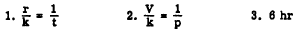Custom SearchVariation as the Power of a Quantity Another form of direct variation occurs when a quantity varies as some power of another. For example,, consider the formula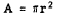Table 13-1 shows the values of r and the corresponding values of A. Table 13-1.-Relation between values of radius and area in a circle.Notice how A changes as a result of a change in r. When r changes from 1 to 2, A changes from p to 4 times p or 22 times p. Likewise when r changes from 3 to 4, A changes not as r, but as the SQUARE of r. In general, one quantity varies as the power of another if it is equal to a constant times that quantity raised to the power. Thus, in an equation such as x = kyn, x varies directly as the nth power of y. As y increases, x increases but more rapidly than y, and as y decreases, x decreases, but again more rapidly. Practice problems. 1. In the formula V = e3, how does V vary? 2. In the formula A = s2, if s is doubled how much is A increased? 3. In the formula s =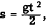is a constant. If t is halved, what is the resulting change in s ? Answers: 1. Directly as the cube of e. 2. It is multiplied by 4. 3. It is multiplied by 1/4. INVERSE VARIATION A quantity VARIES INVERSELY as another quantity if the product of the two quantities is a constant. For example, if x and y are variables and k is a constant, the fact that x varies inversely as y is expressed by         xy=k or         x=- k/y If values are substituted for x and y, we see that as one increases, the other must decrease, and vice versa. Otherwise, their product will not equal the same constant each time. If a quantity varies inversely as a second quantity, it is INVERSELY PROPORTIONAL to the second quantity. In xy = k, the coefficient of k is 1. The equality xy = k can be written in the form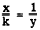or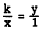Notice that when one of the variables, x or y, occurs in the numerator of a ratio, the other variable occurs in the denominator of the second ratio. This implies that x and y are inversely proportional. Inverse variation may be illustrated by means of the formula for area of a rectangle. If A stands for area, L for length, and W for width, the expression for the area of a rectangle in terms of the length and width is         A = LW Suppose that several rectangles, all having the same area but varying lengths and widths, are to be compared. Then LW = A has the same form as xy = k, where A and k are constants. Thus L is inversely proportional to W, and W is inversely proportional to L. If the constant area is 12 sq ft, this relationship becomes         LW = 12 If the length is 4 ft, the width is found as follows:If the length increases to 6 ft, the width decreases as follows: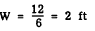If a constant area is 12, the width of a rectangle decreases from 3 to 2 as the length increases from 4 to 6. When two inversely proportional quantities vary, one decreases as the other increases. Another example of inverse variation is found in the study of electricity. The current flowing in an electrical circuit at a constant potential varies inversely as the resistance of the circuit. Suppose that the current, 1, is 10 amperes when the resistance, R, is 11 ohms and it is desired to find the current when the resistance is 5 ohms. Since I and R vary inversely, the equation for the relationship is IR = k, where k is the constant voltage. Therefore, (10)(11) = k. Also, when the resistance changes to 5 ohms, (5)(I) = k. Quantities equal to the same quantity are equal to each other, so we have the following equation: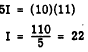The current is 22 amperes when the resistance is 5 ohms. As the resistance decreases from 11 to 5 ohms, the current increases from 10 to 22 amperes. One type of variation problem which tends to be confusing to the beginner involves rates of speed or rates of doing work. For example, if 7 men can complete a job in 20 days, how long will 50 men require to complete the same job? The strictly mechanical approach to this problem might result in the following false solution, relating men to men and days to days: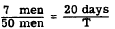However, a little thought brings out the fact that we are dealing with an INVERSE relationship rather than a direct one. In other words, the more men we have, the less time is required. Therefore, the correct solution requires that we use an inverse proportion; that is, we must invert one of the ratios as follows:Practice problems. In problems 1 and 2, express the given data as a proportion, using k as the constant of proportionality. 1. The rate, r, at which a vessel travels in going a certain distance varies inversely as the time, t. 2. The volume, V, of a gas varies inversely as the pressure, p. 3. A ship moving at a rate of 15 knots requires 10 hr to travel a certain distance. If the speed is increased to 25 knots, how long will the ship require to travel the same distance? Answers: